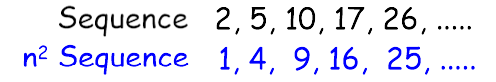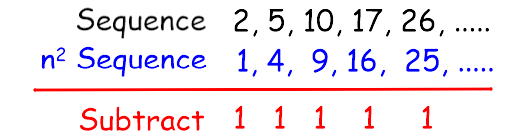# Find the nth Term Rule of a Simple Quadratic Sequence

In this worksheet, students will find the nth term rule of a simple quadratic sequence.Key stage:  KS 3

Curriculum topic:   Algebra

Curriculum subtopic:   Substitute Numerical Values for Formulae/Expressions

Difficulty level:#### Worksheet Overview

This activity is about finding a rule to generate a quadratic sequence.

To find the nth term rule for an arithmetic sequence:

Let's take the sequence 3, 5, 7, 9, ..........

The first difference is +2 as we can see that the next term is 2 more than the previous term.

This means the sequence goes up in the 2 times table!

We call this 2n

However, the first term in the 2 times table is 2 and we want 3, so we have to add 1 to all the terms.

The rule to find any term in that sequence is 2n + 1Let's look at a quadratic sequence now!

What we mean by a quadratic sequence is that it has n in the rule.

Unlike the arithmetic sequence above, it does not increase by the same amount each time!

Let's look at an example!

Find the nth term rule for the quadratic sequence:

2, 5, 10, 17, 26, ............

Method

We look at the nsequence.

That is 12, 22,  32,  42, 52,..........etc

Which is, 1, 4, 9, 16, 25, ..........We can see that the sequence is very similar.

What is the difference?We can see that if we subtract the n2 sequence, the sequence we have is 1 more each time.

Therefore, the rule is n2 + 1It looks a bit complicated, but when you have done a couple, it gets easy very quickly!

Let's give it a go!

### What is EdPlace?

We're your National Curriculum aligned online education content provider helping each child succeed in English, maths and science from year 1 to GCSE. With an EdPlace account you’ll be able to track and measure progress, helping each child achieve their best. We build confidence and attainment by personalising each child’s learning at a level that suits them.

Get started